"The most original and wonderful instrument in scientific history"
Lord Rutherford

## Beta- decay

Beta- rays are composed of electrons. As in alpha rays, they are ejected during the decay of some isotopes

### a.    Potassium

In this equation, one atom of Potassium 40 (with 19 protons and 21 neutrons) undergoes the transformation of a neutron into a proton (and one antineutrino) to become a Calcium atom (20 protons and 20 neutrons):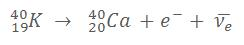In naturally occurring Potassium, about 1 atom every 10 000 is a radioactive isotope of Potassium 40. We each contain a significant amount of Potassium in our body (about 200g) so every second, 5000 atoms of Potassium 40 undergo a radioactive decay. It’s the secondmost natural source of irradiation of people after Radon.

The electron ejected during the decay is a very light particle (9x10-31 kg) with a negative charge; therefore it’s easily deflected by magnetic fields as shown in this image (magnetic field of 0.12 T)Figure 17 : One electron coming from the decay of Potassium 40 being deflected by the magnetic field

The amount of deflection depends on the intensity of the magnetic field and the speed of the particle (its speed is linked to the amount of energy emitted during the decay). By measuring the radius of curvature of the particle, it’s possible to estimate its energy with the help of the graph below. The picture above shows a 3cm radius of curvature which correspond to an energy of 0.7 MeV. Charts of nuclides predict for K40 a maximal energy of 1.3 MeV and a mean energy of 0.56 MeV.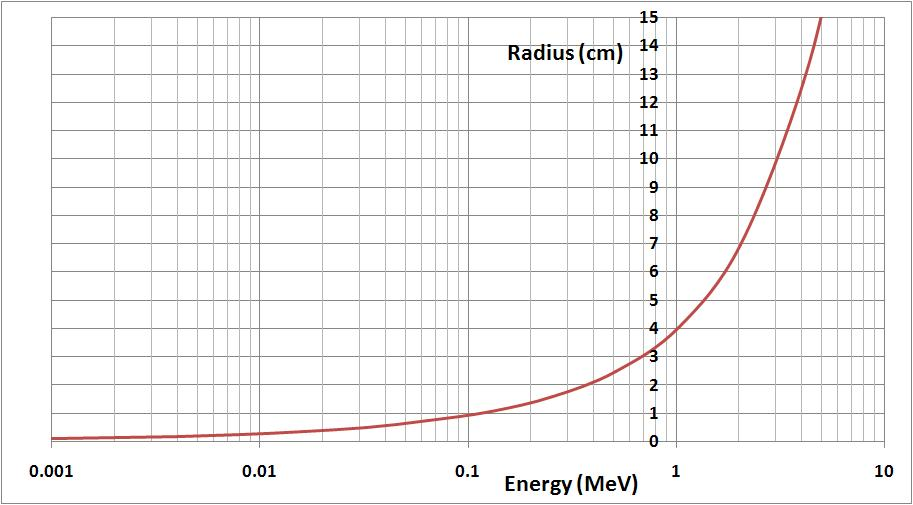Figure 18 : Energy-Radius of curvature equivalence (with relativistic effect considered)

### b.    Uranium

Uranium 238 (also known as “depleted” uranium) is mainly an alpha emitter, but its daughter nuclei also emit a few beta and gamma rays. We can observe those beta rays deflected by the magnetic field in the following image (We can’t see alpha particles which are blocked by the glass of the ampoule):Figure 19 : Electrons emissions by depleted uranium

### c.    Thorium

As in Uranium, Thorium is an alpha and beta- particle emitter, so the effects in the cloud chamber look similar.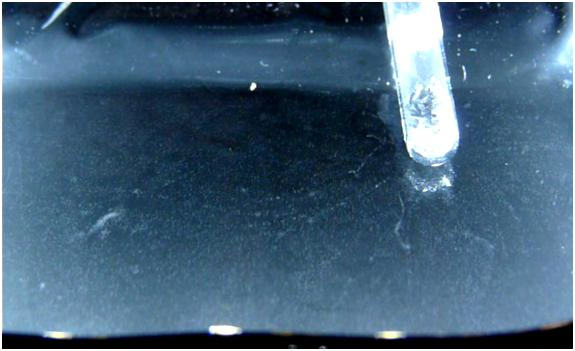Figure 20 : Emission of electrons by thorium

### d.    Trinitite

As well as Alpha particles (see here : alpha particles from Trinitite ), some radioactive isotopes enclosed into Trinitite emits beta particles. Those isotopes are most likely Cesium137 and Strontium90 which are mainly beta emitters.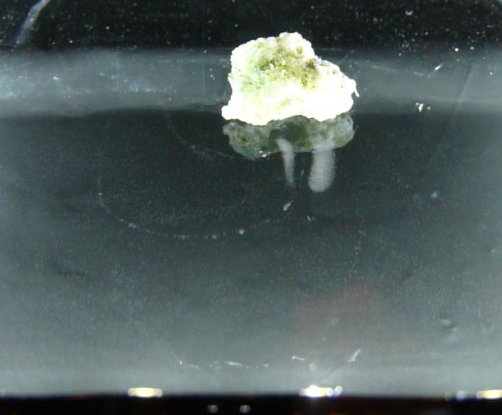Figure 20.1 : Emission of beta particles from Trinitite

## 2.   Beta+ rays

Beta+ rays consist of positrons. A proton is converted to a neutron thanks the weak nuclear force, accordingly a β+ particle (positron) and a neutrino are emitted.
Example of a β+ reaction where Potassium decays into Argon :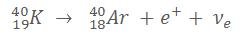Among the radioactive sources in my possession, only Potassium is able to undergo Beta+ decay. Unfortunately, the probability is too low to observe the phenomenon in the cloud chamber (1 atom every 100 000 atoms of K40, so 1 atom every 10 000 000 000 of naturally occurring Potassium).

Edit :
I received a disk calibration source of Na22. Unfortunatly I'm not able to observe the positrons emitted by its beta+ decay. Their lifetime may not be long enough to be distinguished among the electrons (created by gamma rays which interact by photoelectric-effect or compton effect)